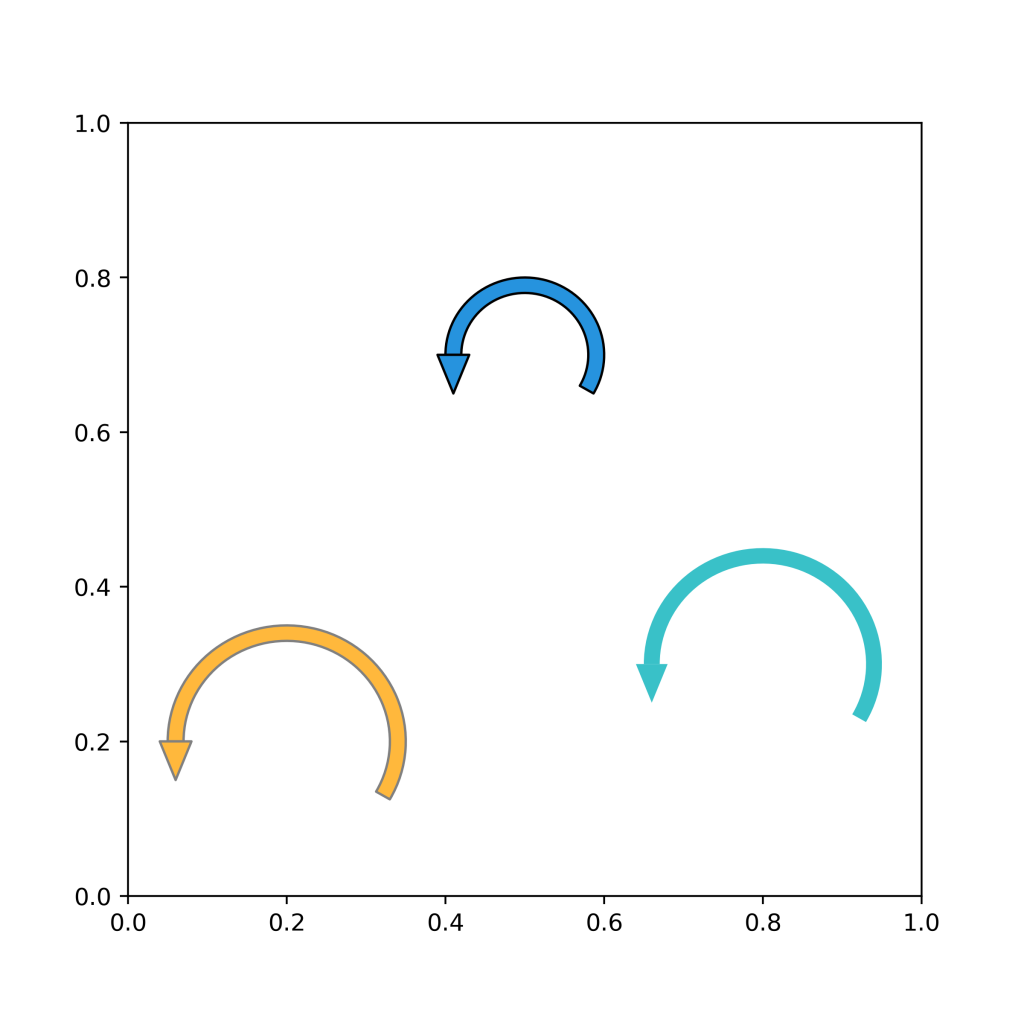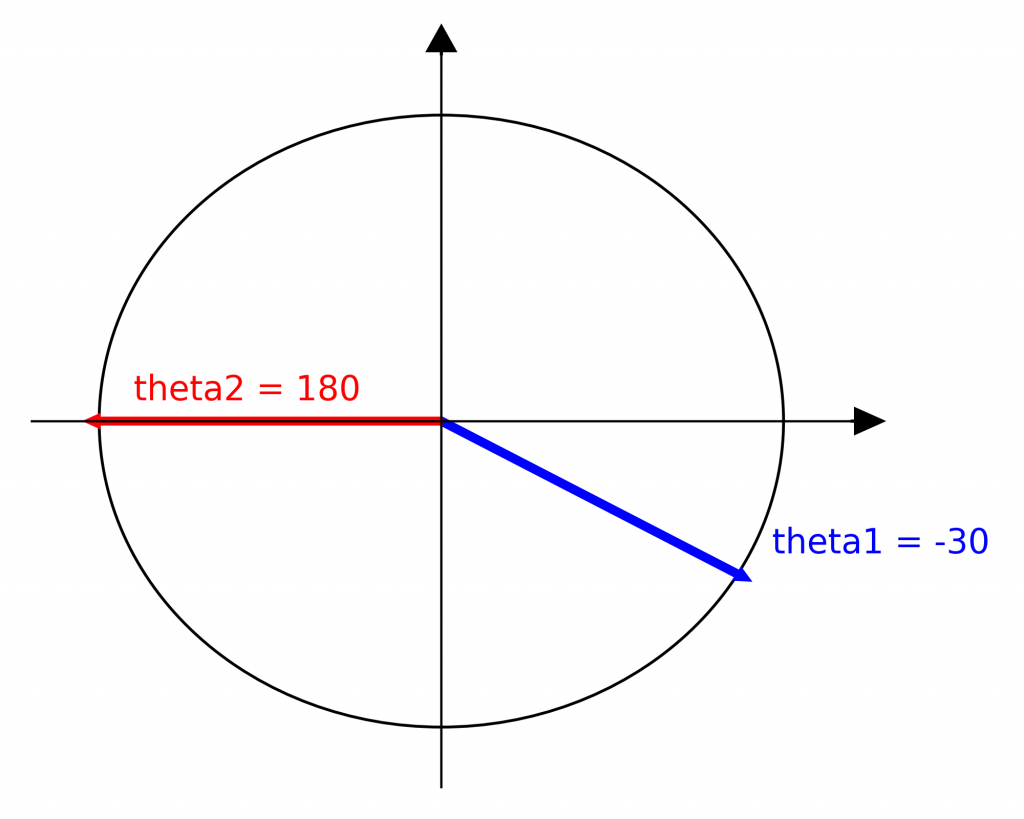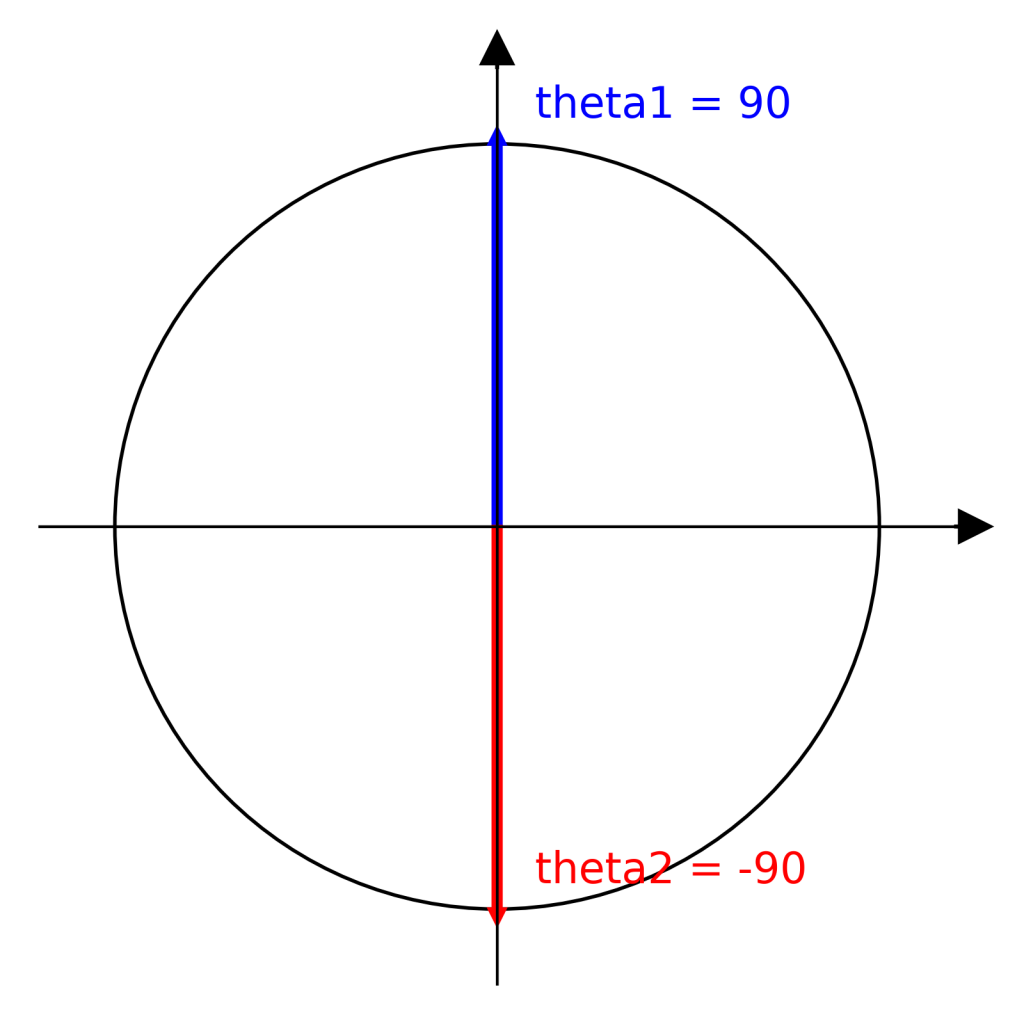# How to draw an arrow that loops with Matplotlib

The function below will be useful if you want to draw an arrow that loops back – a self-loop – in Python.### Full Code

```import numpy as np
import matplotlib.patches as mpatches
from matplotlib.collections import PatchCollection
import matplotlib.pyplot as plt

def draw_self_loop(center, radius, facecolor='#2693de', edgecolor='#000000', theta1=-30, theta2=180):

rwidth = 0.02
ring = mpatches.Wedge(center, radius, theta1, theta2, width=rwidth)

# Triangle edges
offset = 0.02
xcent  = center - radius + (rwidth/2)
left   = [xcent - offset, center]
right  = [xcent + offset, center]
bottom = [(left+right)/2., center-0.05]
arrow  = plt.Polygon([left, right, bottom, left])

p = PatchCollection(
[ring, arrow],
edgecolor = edgecolor,
facecolor = facecolor
)

#---------------------------------------------
# Drawing time
#---------------------------------------------
fig, ax = plt.subplots(figsize=(6,6))
plt.show()
```

### Playing with loop start and loop end

The `mpatches.Wedge` object of `matplotlib.patches` allows you to specify the start and end angles of your loop. You have to visualize these values as situated on a trigonometric circle. In my `draw_self_loop()` function above, the default values are

• theta1 = -30
• theta2 = 180

Which look like this on the trigonometric circleWhich is why your loop looks like this

```fig, ax = plt.subplots(figsize=(4,4))
plt.show()```As you change the valyes of `theta1` and `theta2`, just remember where they would be on the trigonometric circle.

Example

```fig, ax = plt.subplots(figsize=(4,4))The triangle position and direction could be automatically adjusted according to the `theta` values. Feel free to contribute to my code on GitHub.